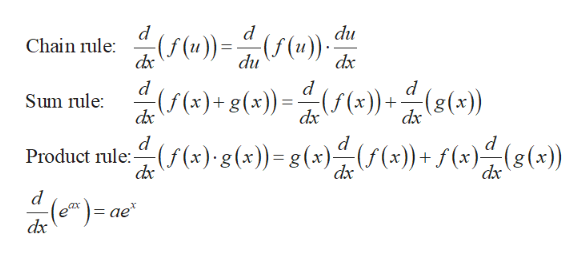# i bozauS1719. y In(e xe)

Question

Differentiate the function

check_circleExpert Solution
Step 1

Recall the following facts ...help_outlineImage Transcriptionclosed du Chain rule:(f(u))=(f(u) du dx )()-)()) (s() (f(x) (f(x)+g(x)) = Sum rule: dx dx d Product rule:f (x) g(x))=g(x)(F(x))+ f(x) dx (e)= ae dx fullscreen

### Want to see the full answer?

See Solution

#### Want to see this answer and more?

Solutions are written by subject experts who are available 24/7. Questions are typically answered within 1 hour*

See Solution
*Response times may vary by subject and question
Tagged in

### Derivative